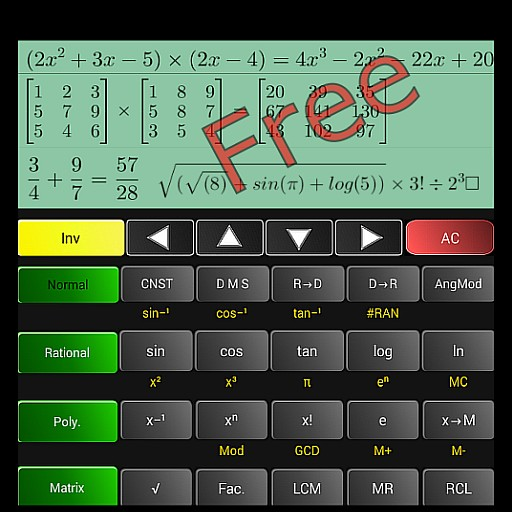## Super Scientific Calculator

### by Tayyeb Amin

Free
4969
Categories Tags
###### Description

Super Scientific calculator is the best free scientific calc with different modes of working, including:
- Natural mathematical expressions calculation
- Polynomial including some algebraic calculation like quadratic equations, evaluation of a polynomial expression and their arithmetic operations
- Rational mode which includes simplification of rational (fractional expressions) and their comparisons along with their arithmetic operations.
- Matrix mode. This includes matrix arithmetic, inverse of matrix, and adjutant of matrix, determinate of matrix, QR decomposition and Transpose.

Display is quite natural along with look and feel of the layout please see screenshots and video.
Salient features include:
1. Writing and evaluation of whole expressions.
2. Use of brackets.
3. LCM (Least Common Multiple), GCD (greatest common divisor).
4. Prime factors of a natural number.
5. Trigonometric functions
7. Memory functions along with history for up to last 100 calculations.
8. nCr, nPr calculation.
9. Powers, roots and logarithms including natural logarithms as well.
10. Result history for natural expressions including Navigations.
11. List of physical constants.
12. Conversion of fractions into decimal numbers.
13. Comparison between two fractions.
14. Arithmetic of two polynomial expressions
And many more.

• AOTD

1570
• AOTD

607

948